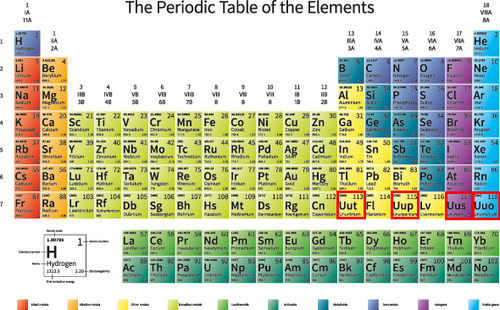Electron Configuration
3 years ago
carreau
Save
Edit
Host a game
Live GameLive
Homework
Solo Practice
Practice20 QuestionsShow answers
• Question 1
300 seconds
Q. What atom matches this electron configuration?
1s2s2p3s2
Neon
Magnesium
Aluminum
Potassium
• Question 2
300 seconds
Q. Which is the electron configuration for an oxygen atom?
1s22s22p63s23p64s2
1s22s22p4
1s22s22p6
1s22s22p63s23p6
• Question 3
300 seconds
Q. What is the maximum number of electrons that an orbital can have?
1 electron
2 electrons
3 electrons
4 electrons
• Question 4
300 secondsQ. How many valence electrons are represented here?
7
5
2
8
• Question 5
300 seconds
Q. How many d orbitals are there in a given sublevel?
1
3
5
7
• Question 6
300 seconds
Q. How many p orbitals are there in a sublevel?
2
1
4
3
• Question 7
300 seconds
Q. How many electrons can the d sublevel hold?
8
10
2
4
• Question 8
300 seconds
Q. What is this element?
1s22s22p63s23p6
4s23d104p6
Argon
Krypton
Selenium
Bromide
• Question 9
300 seconds
Q. What atom matches this electron configuration?
[Xe] 6s2 4f14 5d9
Mercury
Gold
Platinum
Thallium
• Question 10
300 seconds
Q. What is the shorthand electron configuration for Sulfur atom?
[Ar] 3p4
[He] 3s23p4
[Ne] 3s23p4
[Na] 3s23p3
• Question 11
300 seconds
Q. How many electrons can the first energy level hold?
1
2
8
0
• Question 12
300 seconds
Q. How many valence electrons does Chlorine have?
5
2
7
5
• Question 13
300 seconds
Q. How many valence electrons does phosphorus have?
5
2
8
15
• Question 14
300 seconds
Q. What element has the valence shell configuration
3s2 3p2
Al
B
Si
Ga
• Question 15
300 secondsQ. Which element is pictured?
neon
fluorine
magnesium
argon
• Question 16
300 seconds
Q. What noble gas should be used to write the shorthand configuration for Te?
Ar
Kr
Xe
Sb
• Question 17
300 seconds
Q. Identify the element in Period 5 that has 1 valence electron?
Rb
Nb
Ag
Sb
• Question 18
30 seconds
Q. What element in Period 4 has 5 valence electrons?
Zr
As
V
Sb
• Question 19
30 seconds
Q. Which of the following is a p block element?
Ca
Ar
Re
Au
• Question 20
30 seconds
Q. Electron arrangement that uses arrows